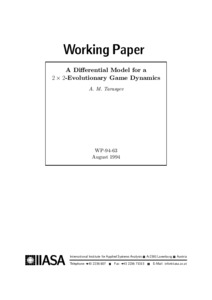# A Differential Model for a 2x2-Evolutionary Game Dynamics

Tarasyev, A.M. (1994). A Differential Model for a 2x2-Evolutionary Game Dynamics. IIASA Working Paper. IIASA, Laxenburg, Austria: WP-94-063Preview Text WP-94-063.pdf Download (2MB) | Preview

## Abstract

A dynamical model for an evolutionary nonantagonistic (nonzero sum) game between two populations is considered. A scheme of a dynamical Nash equilibrium in the class of feedback (discontinuous) controls is proposed. The construction is based on solutions of auxiliary antagonistic (zero-sum) differential games. A method for approximating the corresponding value functions is developed. The method uses approximation schemes for constructing generalized (minimax, viscosity) solutions of first order partial differential equations of Hamilton-Jacobi type. A numerical realization of a grid procedure is described. Questions of convergence of approximate solutions to the generalized one (the value function) are discussed, and estimates of convergence are pointed out. The method provides equilibrium feedbacks in parallel with the value functions. Implementation of grid approximations for feedback control is justified. Coordination of long- and short-term interests of populations and individuals is indicated. A possible relation of the proposed game model to the classical replicator dynamics is outlined.

Item Type: Monograph (IIASA Working Paper) Dynamic Systems (DYN) IIASA Import 15 Jan 2016 02:04 27 Aug 2021 17:14 https://pure.iiasa.ac.at/4148View Item